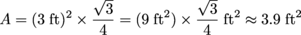## Area of Triangles

When we first started looking at shapes, we began with the simplest possible 2D shape: the triangle. Why? Well, because it was the simplest to handle. Though you probably know by now that those three sides can pack a wallop. After all, we spent three whole sections on them, didn't we?

Don't worry. Compared to proving congruence and finding sines and cosines, finding a triangle's area is like a trip down Easy Street. The area of a triangle can be calculated with the simple formula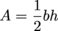,

where A is the area, b is the base, and h is the height. And, quite obviously, one half is one half. Area is half the base times the height. That's all there is to it.

### Sample Problem

Find the area of a triangle with a base of 6 inches and a height of 8 inches.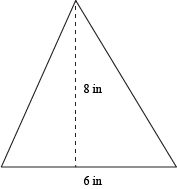Applying the formula for the area of a triangle,, all we have to do is plug in our values for the base and the height, which are 6 inches and 8 inches.

A = ½(6 in)(8 in)
A = 24 in2

Okay, fine. So maybe it's not always that easy. After all, what is a base? What is a height? What is a half? (One half is still one half. That hasn't changed.)

The base can be any side of the triangle, but the height depends on which side we use as the base.

This makes sense in the non-math world too. Harrison Ford's height might be 6'1" when standing up, but when he's lying down on the floor, cowering in fear from Darth Vader, his height from the ground might only be 1 foot. It doesn't mean he shrank. It just means we're using a different base.The height of a triangle is defined as the perpendicular distance to the base from the vertex opposite the base. In other words, you know how a triangle has three sharp looking points? Take the one opposite your base. Then draw a perpendicular line from that point to your base, even if it means extending your base.As you might imagine, right triangles are our best friends, because two of the sides already are perpendicular. So one of the legs is the base, and the other is automatically the height. Maybe we should make some more three-dimensional friends…

### Sample Problem

What is the area of the triangle below?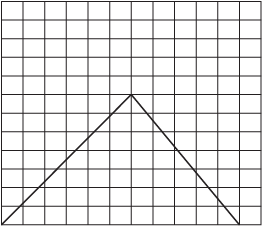The triangle here has a base of 11 units and a height of 7 units. (Our handy-dandy grid told us that.) So we can plug those values into the formulato find the triangle's area.

A = ½(11 units)(7 units)
A = 38.5 units2

In other words, there are 38 and a half square units inside of the triangle. We can also think the triangle as being pregnant with 38.5 little square babies. Uh…why would a triangle have square babies? We smell adultery.

### Sample Problem

A triangle has an area of 12 ft2 and a base that's 48 inches long. What is the height of the triangle?

Hopefully, the first thing you noticed was that the units are different. Since there are 12 inches in a foot (and 10 toes, usually), we can convert the base into feet by dividing by 12. This gives us 4 ft as the length of the base. Then, we can rearrange the triangular area formula to solve for height and plug in the values A = 12 ft2 and b = 4 ft.

A = ½bh
h = 2Ab
h = 2(12)4
h = 6 ft

What if we had wanted to convert the area to inches instead of the base to feet? We can certainly do that. It's just 144 inches, right? After all, there are 12 inches in a foot, so 12 × 12 = 144.

No! Don't do it! It's a trap!

The area of the triangle is not 12 feet. It's 12 square feet remember? So 1 foot may equal 12 inches, but 1 square foot is equal to 144 square inches. (It's because 1 foot by 1 foot is the same as 12 inches by 12 inches.)

If you're unsure, you can also convert using the "normal" one-dimensional conversion, and square the number for every additional dimension you need.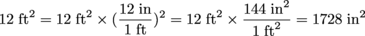Now, we can continue the problem using b = 48 inches and A = 1728 inches2.

h = 2Ab
h = 2(1728)48
h = 72 in

Yep, that's the same answer we got before: h = 6 feet, which is the same thing as 72 inches.

Just in case you can't get enough of formulas, there's another one that you might find useful. Rather than working hard to find the height of an equilateral triangle, we can calculate its area based on the length of the side alone. For any equilateral triangle with side s, its area A can be found using the formula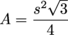Pretty nifty, ain't it?

### Sample Problem

Find the area of an equilateral triangle whose sides are all 3 feet long.

It's really a plug-and-chug sort of situation, here. All we need to do is substitute 3 ft for the s in the formula, and hope our calculator and arithmetic skills are up to snuff.